PHY294H - Lecture 16

Current and voltage dividers

A useful way of thinking about series and parallel resistor combinations is as voltage and current dividers. If two resistors R1 and R2 are connected in series, and an emf,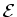, is applied to the series combination, then the voltages across R1 and R2 obey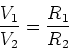(1)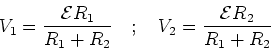(2)

Clearly the largest part of the emf is dissipated in the resistance which is largest.

A parallel combination of resistors R1 and R2 divides the current according to,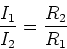(3)

In this case the smallest of the resistors carries the most current.

Wheatstone bridge examples

Consider five resistors in a wheatstone bridge geometry. The top two resistors are R1 and R2, while the bottom two resistors are R4 and R5. The center resistor is R3. If the applied source has emf, write down all of the possible junction equations and the loop equations for the circuit. Some things to note

- It is hard to solve this analytically. Series and parallel combinations of resistors is not enough.

- The special case where I3 = 0 is important. When this is true R2/R4 = R1/R5. This provides a very sensitive way to measure an unknown resistance.

- If we set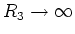, there may still be a voltage across R3. This voltage is given by,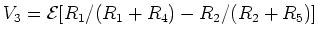.

Ammeters and voltmeters

There are devices called galvanometers which measure the amount of current in a circuit. Most used to be based on the principle that when magnetic field is applied to a coil which carries a current, the coil experiences a torque. The torque provides motion to a needle which provides a measure of the current. We shall return to this device when we analyse the interactions between currents and magnetic fields later in the course. These days currents are measured using solid state current detectors and the output is digitized. For the moment we assume that galvanometers have small internal resistance and are very sensitive current detectors. Galvanometers are the heart and soul of both ammeters and voltmeters.

Ammeters measure current and voltmeters measure voltage. Multimeters can measure either voltage or current. Any measuring device should strive to have as little effect as possible on the circuit which it measures. Ammeters are placed in series with the circuit element across which we want to measure the current. They have very small internal resistance so as to minimize the amount of voltage dropped across them. In contrast voltmeters are placed in parallel with the circuit that we want to measure so they should have very high internal resistance.

We also worked problems FGT 28.32, 28.40.